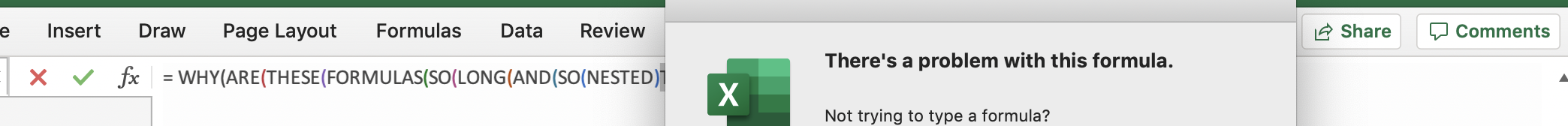So so long

# Some formulas to make working in Excel easier

If you’re used to programming in R or Python and suddenly have to do everything in Excel due to client requirements or something, you may repeatedly find yourself wondering “How do I do this thing I always do? Shouldn’t it be easy?”. Well the answer likely is no, it isn’t as easy as it should be, but yes, there is a way. Here’s a few of them that I couldn’t google for that easily.

Some of the cell array formulas only work in Office365 - for older versions of Excel you’ll have to do some ctrl-shift-enter faffing around to make the cell array formulas work.

# Checking a cell for the presence of one of multiple partial text string matches

Say the data has been entered really non-uniformly. Sometimes it says ‘Yes’, sometimes it’s a 1 etc. like in the following table:

Yes valueNo valueMissing data
YesNon/a
Yes (unneccesary details)No (unneccesary details)-
10
I think soNot this timeNA

Any cell can contain one of these options and the unnecesary details might change. So really you want to search for a list of items and if any appear, mark it as true.

Example code to search any of the ‘Yes’ values and recode them as TRUE:

= OR( NOT( ISERROR( SEARCH({ "Yes" , "1" , "I think so" } , cell_to_search ) ) ) )


How does it work?

1. This is feeding a cell array of possible yes values { "Yes" , "1" , "I think so" } into the search function along with the cell to search. This will output a cell array which contains a 1 where it matched the search string, and a #VALUE error where it didn’t, for example if the cell contents was “Yes blah”, it will return {1, #VALUE, #VALUE}
2. The ISERROR() function will turn this into true/false values e.g. {FALSE, TRUE, TRUE}
3. The NOT() function negates it to {TRUE, FALSE, FALSE}
4. The OR() function concatenates it appropriately to a single TRUE value output.

You can then nest some if statements to match both yes and no, leaving everything else as missing.

# Finding the unique elements in an array

If you want to find the unique elements in a column and return them sorted, it’s easy. Just use =SORT(UNIQUE(cells)). However if you want to do this for values occuring across multiple columns in an array, it’s annoying.

First, select your array and give it a name, e.g. MyArray. Then, use this horrible formula:

=SORT(UNIQUE(INDEX(MyArray,1+INT((ROW(INDIRECT("1:"&COLUMNS(MyArray)*ROWS(MyArray)))-1)/COLUMNS(MyArray)),MOD(ROW(INDIRECT("1:"&COLUMNS(MyArray)*ROWS(MyArray)))-1+COLUMNS(MyArray),COLUMNS(MyArray))+1)))

How does it work? Lets break it into parts:

1. Array element iterator ROW(INDIRECT("1:"&COLUMNS(MyArray)*ROWS(MyArray))). Using the formula =ROW(INDIRECT("1:10")) will give me a cell array of {1;2;3;4;5;6;7;8;9;10}. So to get an iterator to go through all the elements in the array, I use ROW(INDIRECT("1:"&COLUMNS(MyArray)*ROWS(MyArray))). Let’s call this iterator i now.
2. Indexing the array INDEX(array, row, column) will pull out an element of the array. so we do appropriate modulo arithmatic on the iterator i to get the column number - MOD(i-1+COLUMNS(MyArray),COLUMNS(MyArray))+1, and take the floor of a division (using the INT() function) to get the row number as row = 1+INT((i-1)/COLUMNS(MyArray)). So we get INDEX(MyArray,1+INT((i-1)/COLUMNS(MyArray)),MOD(i-1+COLUMNS(MyArray),COLUMNS(MyArray))+1)
3. Now we have a vertical array/column (let’s call it col ) containing all the data which was in the array. We just have to apply SORT(UNIQUE(col)) to get out only the unique elements, and sorted in alphabetical order.

# My mind went blank

1. Referencing the contents of a cell will return the contents of the cell, unless it is blank. A blank cell will turn into a zero. Thus missing data can magically turn into non-missing data in the middle of your analysis. Instead of =cell_ref use =if(isblank(cell_ref),"",cell_ref) to keep blanks blank.Blanks can sneakily turn into the number zero
2. Checking if a cell is blank using isblank(cell_ref) will return false if the cell contains a formula which returns a blank string as a value. In this case use cell_ref="" instead.Blanks can sneakily avoid detection if they are the result of a formula

# Examples

In the file excel_formulas_example.xlsx are examples of the things described above.